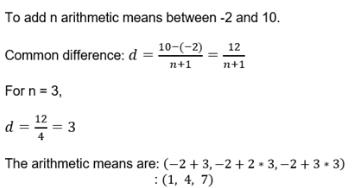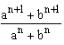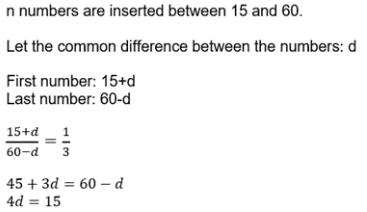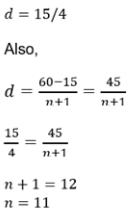Courses

Test: Arithmetic Mean

10 Questions MCQ Test Mathematics For JEE | Test: Arithmetic Mean

Description
Attempt Test: Arithmetic Mean | 10 questions in 10 minutes | Mock test for JEE preparation | Free important questions MCQ to study Mathematics For JEE for JEE Exam | Download free PDF with solutions
QUESTION: 1

The three arithmetic mean between – 2 and 10 are:

Solution:QUESTION: 2

If a, b, c are in A.P., then

Solution:

If a, b, c are in A.P., then b is the arithmetic mean of a and c.
a b and c are in AP.
∴ b - a = c - b
⇒ 2b = a + c          ........ (1)
The arithmetic mean of a and c
= (a+c)/2
Using equation (1), we get
= 2b/2
= b
∴ The arithmetic mean of a and c = b
Thus, the arithmetic mean of a and c is b.

QUESTION: 3

If we want to insert 8 numbers between the numbers 4 and 31 such that the resulting sequence is an AP.The difference between the consecutive numbers will be

Solution:

a = 4, l = 31, n = 8
(l-a)/(n+1) = (31-4)/(8+1)
= 27/9
= 3

QUESTION: 4

For what value of n,is the arithmetic mean (AM) of a and b?

Solution:

(aⁿ⁺¹ + bⁿ⁺¹)/(aⁿ + bⁿ) is mean between a & b
Mean of a & b =  ( a + b)/2
=> (aⁿ⁺¹ + bⁿ⁺¹)/(aⁿ + bⁿ) = ( a + b)/2
=> 2aⁿ⁺¹ + 2bⁿ⁺¹  = aⁿ⁺¹  + bⁿ⁺¹  + baⁿ + bⁿa
=> aⁿ⁺¹ + bⁿ⁺¹ = baⁿ + bⁿa
=> aⁿ(a - b) = bⁿ(a - b)
=> aⁿ = bⁿ
=> (a/b)ⁿ = 1
=> n = 0    or a  = b

QUESTION: 5

The digits of a positive integer having three digits are in AP and sum of their digits is 21. The number obtained by reversing the digits is 396 less than the original number. Find the original number.

Solution:

Let the digits at ones, tens and hundreds place be (a−d)a and (a+d) respectively. The, the number is
(a+d)×100+a×10+(a−d) = 111a+99d
The number obtained by reversing the digits is
(a−d)×+a×10+(a+d) = 111a−99d
It is given that the sum of the digits is 21.
(a−d)+a+(a+d) = 21                        ...(i)
Also it is given that the number obtained by reversing the digits is 594 less than the original number.
∴111a−99d = 111a+99d−396          ...(ii)
⟹ 3a = 21 and 198d = 396
⟹ a = 7 and d = -2
Original number = (a−d)×+a×10+(a+d)
= 100(9) + 10(7) + 5
= 975

QUESTION: 6

A man saved Rs 21700 in 14 years. In each year after the first he saved Rs 100 more than he did in the preceding year. How much did he save in the first year?

Solution:

d = 100
Sn = 21700
n = 14
Sn = n/2[2a + (n-1)d]
21700 = 14/2[2a + (14-1)100]
= 21700 = 7[2a + 1300]
= 3100 = 2a + 1300
⇒ 3100 - 1300 = 2a
⇒ 1800 = 2a
⇒ a = 900

QUESTION: 7

What is the 10th A.M between 2 and 57 if 10 A.M s are inserted between these numbers?

Solution:

an = 57, a = 2, n = 12
an = a + (n-1)d
=> 57 = 2 + (12 - 1)d
=> 55 = 11d
d = 5
T10 = a + 10d
=> 2 + 10(5)
= 52

QUESTION: 8

If n numbers are inserted between 15 and 60 such that the ratio of the first to the last is 1 : 3, then the value of n is:

Solution:QUESTION: 9

The arithmetic mean between 6 and – 12 is:

Solution:

6, x, -12
x = (6-12)/2
x = -6/2
x = -3

QUESTION: 10

If A1, A2, A3,…., An are n numbers between a and b, such that a, A1, A2, A3,…, An, b are in A.P., then nth term from beginning is:

Solution:

A1,A2,......, An are inserted between a and b then the series will become
a, A1,A2,A3,......, An,b. Now a becomes the first term, A1 will be second, A2 will become third term
An will become A(n+1)th term
therefore A(n-1) will become nth term.Use Code STAYHOME200 and get INR 200 additional OFF Use Coupon Code

Track your progress, build streaks, highlight & save important lessons and more!

Similar ContentRelated tests